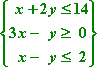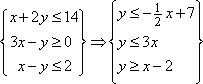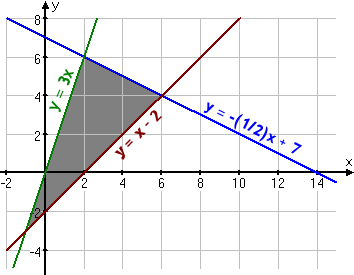Search

Linear Programming: Introduction (page 1 of 5)

Sections: Optimizing linear systems, Setting up word problemsLinear programming is the process of taking various linear inequalities relating to some situation, and finding the "best" value obtainable under those conditions. A typical example would be taking the limitations of materials and labor, and then determining the "best" production levels for maximal profits under those conditions.

In "real life", linear programming is part of a very important area of mathematics called "optimization techniques". This field of study (or at least the applied results of it) are used every day in the organization and allocation of resources. These "real life" systems can have dozens or hundreds of variables, or more. In algebra, though, you'll only work with the simple (and graphable) two-variable linear case.

The general process for solving linear-programming exercises is to graph the inequalities (called the "constraints") to form a walled-off area on the x,y-plane (called the "feasibility region"). Then you figure out the coordinates of the corners of this feasibility region (that is, you find the intersection points of the various pairs of lines), and test these corner points in the formula (called the "optimization equation") for which you're trying to find the highest or lowest value.

• Find the maximal and minimal value of z = 3x + 4y subject to the following constraints:
•The three inequalities in the curly braces are the constraints. The area of the plane that they mark off will be the feasibility region. The formula "z = 3x + 4y" is the optimization equation. I need to find the (x, y) corner points of the feasibility region that return the largest and smallest values of z.

My first step is to solve each inequality for the more-easily graphed equivalent forms:To find the corner points -- which aren't always clear from the graph -- I'll pair the lines (thus forming a system of linear equations) and solve:

 y = ( 1/2 )x + 7y = 3x y = ( 1/2 )x + 7y = x  2 y = 3xy = x  2 ( 1/2 )x + 7 = 3xx + 14 = 6x14 = 7x2 = x y = 3(2) = 6 ( 1/2 )x + 7 = x  2x + 14 = 2x  418 = 3x6 = x y = (6)  2 = 4 3x = x  22x = 2x = 1 y = 3(1) = 3 corner point at (2, 6) corner point at (6, 4) corner pt. at (1, 3)

So the corner points are (2, 6), (6, 4), and (1, 3).

Somebody really smart proved that, for linear systems like this, the maximum and minimum values of the optimization equation will always be on the corners of the feasibility region. So, to find the solution to this exercise, I only need to plug these three points into "z = 3x + 4y".

(2, 6):      z = 3(2)   + 4(6)   =   6 + 24 =   30
(6, 4):
z = 3(6)   + 4(4)   = 18 + 16 =   34
(1, 3):  z = 3(1) + 4(3) = 3  12 = 15

Then the maximum of z = 34 occurs at (6, 4),
and
the minimum of z = 15 occurs at (1, 3).

Top  |  1 | 2 | 3 | 4 | 5  |  Return to Index  Next >>

 Cite this article as: Stapel, Elizabeth. "Linear Programming: Introduction." Purplemath. Available from     https://www.purplemath.com/modules/linprog.htm. Accessed [Date] [Month] 2016

Study Skills Survey

Tutoring from Purplemath
Find a local math tutor